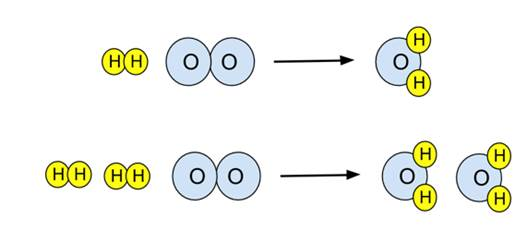# Enduring Understanding 3.A.1: Balanced Chemical Equations

• Chemical equations are used to describe the rearrangement of atoms and electrons during a chemical reaction.
• Because atoms can be neither created nor destroyed, and always react in fixed proportions, each side of the equation must contain the same number of each kind of atom. This is called a balanced equation.

• Example: For the reaction of hydrogen and oxygen to give water,
• H2 + O2 → H2O
• This equation is unbalanced. There are two oxygens on the reactant side, only one on the product side. To balance it, the number of product water molecules must be increased to 2, and then the number of reactant H2 molecules must also be increased to 2. This provides the balanced equation:
• 2H2 + O2 → 2H2O
• This reaction can be illustrated in a particulate diagram. The unbalanced equation on top, the balanced equation below.
•• Note the upper diagram is not balanced - there are a different number of oxygen atoms on either side of the arrow. The lower equation is balanced.

 Related Links: Chemistry Chemistry Quizzes AP Chemistry Notes Lewis Structures and VSEPR AP Chemistry Quizzes Balancing Chemical Equations Worksheets Atoms, Molecules, and Chemical Analysis Quiz Electronic Structure of Atoms Quiz Periodicity, Atomic & Ionic Radii, Atomic Models Quiz Conservation of Matter and Balanced Equations Quiz Gases and Solutions Quiz Intermolecular Forces Quiz Molecular Geometry and VSEPR Quiz Bonding and Solid State Properties Quiz Balanced and Ionic Equations Quiz Chemical Reactions Quiz Redox Reactions and Electrochemistry Quiz Chemical Kinetics and Rates Quiz Reaction Rates and Collisions Quiz Multi-Step Reaction Rates and Catalysis Quiz Energy and Heat Quiz Conservation of Energy and Calorimetry Quiz Bond Energies Quiz Entropy and Gibbs Free Energy Quiz Chemical Equilibrium Quiz Solubility and Acid-Base Equilibrium Quiz

To link to this Balanced Chemical Equations page, copy the following code to your site: